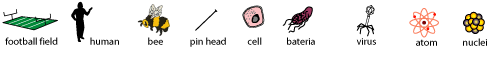# Exponents and Scientific Notation

Exploring Math and Science#### Introduction

The exciting connections between mathematics and science are endless. Scientists from many fields use mathematics to express relationships and to measure the size and distance of objects. You have been selected to explore some of these connections.

To prepare for these explorations, you will need to review some topics in mathematics including exponents. You will then extend that review by learning about scientific notation. Next, you will demonstrate your understanding of these topics by completing a worksheet on scientific notation with problems from the field of science. You will then do independent online research to gather scientific facts. Lastly, you will create your own problems using the facts you gathered to apply what you learned. These tasks will require a computer, access to the web, paper and pencil.

#### The Process

Be sure to read through all of the directions in each section before you click and follow the links and instructions.

Process Part I: Review

1. Browse our lesson on Multiplying Decimals and Whole Numbers.
2. Review multiplying and dividing decimals by powers of ten in Problem 1 from the lesson above.
3. Review our lesson on Exponents.
4. Complete all five exercises at the end of the lesson.

Process Part II: Extend Your Knowledge

1. Learn about Negative Exponents (external site).
3. Learn about Scientific Notation (external site).
4. Complete our Worksheet on Scientific Notation (PDF).

Scientific notation is used to express very large or very small numbers. A number in scientific notation is written as the product of a number greater than or equal to one and less than ten, and a power of 10. The number between one and ten is called the coefficient, and can be an integer or a decimal. Below are some examples.

57,200,000 = 5.72 x 107
0.000 000 006 1 = 6.1 x 10-9

1. Print the answer key for our Worksheet on Scientific Notation (PDF).

Process Part III: Research To Gather Scientific Facts

Research the following topics below from the field of science. Gather ten scientific facts about these objects using the Internet. The data you gather will be very large and very small numbers.

1. The distance in km from the sun to each planet.
2. The distance in km from any planet to its moon.
3. The half-life of certain radioactive elements.
4. The size of bacteria and viruses.
5. The size of an atom, nucleus or cell.
6. The mass of an electron.

Process Part IV: Apply Your Knowledge

1. Create your own worksheet on scientific notation from the data you have gathered. Your worksheet will consist of ten problems. You must include problems with positive exponents, and problems with negative exponents.
2. Create an answer key for your worksheet (i.e., work out each problem and record the answer).
3. Share your problems with a classmate or family member.

#### Resources

1. Math Goodies lesson on Multiplying Decimals and Whole Numbers.
2. Math Goodies lesson on Exponents.
3. Free Math Help's Solving Negative Exponents.
4. Math Goodies Worksheet on Scientific Notation (PDF).
5. Math Goodies answer key for Worksheet on Scientific Notation (PDF).
6. Science websites through independent online searches. Here are some sites to get you started:

#### Evaluation

1. Print the answer key for our Worksheet on Scientific Notation (PDF) from Process Part II.
3. Have a classmate or family member complete the worksheet you created.